• 一、闭包求法 、 二、求闭包示例 ( 关系图角度 ) 、 三、求闭包示例 ( 关系矩阵角度 ) 、 四、闭包运算与关系性质 、 五、闭包复合运算


文章目录
一、闭包求法二、求闭包示例 ( 关系图角度 )三、求闭包示例 ( 关系矩阵角度 )四、闭包运算与关系性质五、闭包复合运算

一、闭包求法

R

R

关系是

A

A

集合上的二元关系 ,

R

⊆

A

R \subseteq A

, 且

A

A

集合不为空集 ,

A

≠

∅

A \not= \varnothing

求自反闭包 :

r

(

R

)

=

R

∪

I

A

r(R) = R \cup I_A

, 给每个顶点添加环 ;
如果

R

R

关系是自反的 , 当且仅当 ,

I

A

⊆

R

I_A \subseteq R

求对称闭包 :

s

(

R

)

=

R

∪

R

−

1

s(R) = R \cup R^{-1}

原来 没有有向边 ( 有序对 ) , 自然也没有对应的逆 , 此时不添加边原来 有一条有向边 ( 有序对 ) , 再添加一个反向的有向边 , 组成 关系图中的 顶点间的 双向有向边原来 有两条有向边 ( 有序对 ) , 此时就不用添加其它边如果

R

R

关系是对称的 , 当且仅当 ,

R

=

R

−

1

R = R^{-1}

求传递闭包 :

t

(

R

)

=

R

∪

R

2

∪

R

3

∪

⋯

t(R) = R \cup R^2 \cup R^3 \cup \cdots

将

R

R

关系所有的幂运算值并起来 , 就是其传递闭包 ,

R

R

关系的

1

1

次幂 ,

R

R

关系的

2

2

次幂 ,

R

R

关系的

3

3

次幂 ,

⋯

\cdots

,

R

R

关系的

n

n

次幂 , 并起来 , 就是其传递闭包 ;
如果

A

A

是有穷集 , 其关系也是有穷的 , 求出其所有的

n

n

次幂 , 不用求出很多幂运算 , 因为关系的幂运算后面都是循环的 , 求出已知的所有

n

n

次幂 取 并集即可 ;
如果

R

R

关系是传递的 , 当且仅当 ,

R

2

⊆

R

R^2 \subseteq R

二、求闭包示例 ( 关系图角度 )

集合

A

=

{

a

,

b

,

c

,

d

}

A = \{ a, b, c , d \}

关系

R

=

{

<

a

,

b

>

,

<

b

,

a

>

,

<

b

,

c

>

,

<

c

,

d

>

}

R = \{ <a,b> , <b,a> , <b,c> , <c,d> \}

求关系

R

R

的自反闭包

r

(

R

)

r(R)

, 对称闭包

s

(

R

)

s(R)

, 传递闭包

t

(

R

)

t(R)求自反闭包 : 就是给每个顶点加上环 :求对称闭包 : 将 顶点间 单向边改成双向边 , 不管 顶点间双向边 和 顶点间没有边 的情况 ;求传递闭包 : 将能到的点直接连起来 ;
a 可以到 b , 路径 a -> b ; a 可以到 c , 路径是 a -> b -> c ; a 可以到 d , 路径是 a -> b -> c -> d ; 因此添加 a 到 c , d 的有向边 ;b 可以到 a , 路径 b -> a ; b 可以到 c , 路径是 b -> c ; b 可以到 d , 路径是 b -> c -> d ; 因此添加 b 到 d 的有向边 ;c 可以到 d , 路径 c -> d ; 没有可连接的边 ;d 哪都到不了 , 没有可连接的边 ;另外出现双向边时 , 两个顶点必须加环 ;三、求闭包示例 ( 关系矩阵角度 )

关系

R

=

{

<

a

,

b

>

,

<

b

,

a

>

,

<

b

,

c

>

,

<

c

,

d

>

}

R = \{ <a, b> , <b,a> , <b,c> , <c,d> \}

使用关系矩阵方法求其 自反闭包 , 对称闭包 , 传递闭包 ;

将上述关系写成矩阵形式为 :

M

(

R

)

=

[

0

1

0

0

1

0

1

0

0

0

0

1

0

0

0

0

]

M(R) = \begin{bmatrix} 0 & 1 & 0 & 0 \\\\ 1 & 0 & 1 & 0 \\\\ 0 & 0 & 0 & 1 \\\\ 0 & 0 & 0 & 0 \end{bmatrix}

自反闭包 : 将主对角线值 , 全部改成

1

1

, 左上角到右下角为主对角线 ;

M

(

r

(

R

)

)

=

[

1

1

0

0

1

1

1

0

0

0

1

1

0

0

0

1

]

M(r(R)) = \begin{bmatrix} 1 & 1 & 0 & 0 \\\\ 1 & 1 & 1 & 0 \\\\ 0 & 0 & 1 & 1 \\\\ 0 & 0 & 0 & 1 \end{bmatrix}

对称闭包 : 主对角线两端要对称 , 以对角线为基准 , 使对角线两边的值对称 ;

M

(

s

(

R

)

)

=

[

0

1

0

0

1

0

1

0

0

1

0

1

0

0

1

0

]

M(s(R)) = \begin{bmatrix} 0 & 1 & 0 & 0 \\\\ 1 & 0 & 1 & 0 \\\\ 0 & 1 & 0 & 1 \\\\ 0 & 0 & 1 & 0 \end{bmatrix}

传递闭包 : 求该关系矩阵的 二次幂 , 三次幂 , 四次幂 ,

⋯

\cdots

, 直到出现相同的循环的值为止 ;
将上述所有的不同的 矩阵幂运算 进行逻辑相加 ( 或 ) 操作 , 就是其传递闭包对应的矩阵 , 计算机算法适合使用该方法 , 如果人计算 , 还是关系图比较形象 ;
参考 : 【集合论】关系表示 ( 关系矩阵 | 关系矩阵示例 | 关系矩阵性质 | 关系矩阵运算 | 关系图 | 关系图示例 | 关系表示相关性质 ) 四、关系矩阵运算
注意逆序合成

M

(

R

2

)

=

M

(

R

∘

R

)

=

M

(

R

)

∙

M

(

R

)

=

[

0

1

0

0

1

0

1

0

0

0

0

1

0

0

0

0

]

∙

[

0

1

0

0

1

0

1

0

0

0

0

1

0

0

0

0

]

=

[

1

0

1

0

0

1

0

1

0

0

0

0

0

0

0

0

]

M(R^2) = M(R \circ R) = M(R) \bullet M(R) =\begin{bmatrix} 0 & 1 & 0 & 0 \\\\ 1 & 0 & 1 & 0 \\\\ 0 & 0 & 0 & 1 \\\\ 0 & 0 & 0 & 0 \end{bmatrix} \bullet \begin{bmatrix} 0 & 1 & 0 & 0 \\\\ 1 & 0 & 1 & 0 \\\\ 0 & 0 & 0 & 1 \\\\ 0 & 0 & 0 & 0 \end{bmatrix} = \begin{bmatrix} 1 & 0 & 1 & 0 \\\\ 0 & 1 & 0 & 1 \\\\ 0 & 0 & 0 & 0 \\\\ 0 & 0 & 0 & 0 \end{bmatrix}

M

(

R

3

)

=

M

(

R

2

∘

R

)

=

M

(

R

)

∙

M

(

R

2

)

=

[

0

1

0

0

1

0

1

0

0

0

0

1

0

0

0

0

]

∙

[

1

0

1

0

0

1

0

1

0

0

0

0

0

0

0

0

]

=

[

0

1

0

1

1

0

1

0

0

0

0

0

0

0

0

0

]

M(R^3) = M(R^2 \circ R) = M(R) \bullet M(R^2) = \begin{bmatrix} 0 & 1 & 0 & 0 \\\\ 1 & 0 & 1 & 0 \\\\ 0 & 0 & 0 & 1 \\\\ 0 & 0 & 0 & 0 \end{bmatrix} \bullet \begin{bmatrix} 1 & 0 & 1 & 0 \\\\ 0 & 1 & 0 & 1 \\\\ 0 & 0 & 0 & 0 \\\\ 0 & 0 & 0 & 0 \end{bmatrix} = \begin{bmatrix} 0 & 1 & 0 & 1 \\\\ 1 & 0 & 1 & 0 \\\\ 0 & 0 & 0 & 0 \\\\ 0 & 0 & 0 & 0 \end{bmatrix}

M

(

R

4

)

=

M

(

R

3

∘

R

)

=

M

(

R

)

∙

M

(

R

3

)

=

[

0

1

0

0

1

0

1

0

0

0

0

1

0

0

0

0

]

∙

[

0

1

0

1

1

0

1

0

0

0

0

0

0

0

0

0

]

=

[

1

0

1

0

0

1

0

1

0

0

0

0

0

0

0

0

]

=

M

(

R

2

)

M(R^4) = M(R^3 \circ R) = M(R) \bullet M(R^3) =\begin{bmatrix} 0 & 1 & 0 & 0 \\\\ 1 & 0 & 1 & 0 \\\\ 0 & 0 & 0 & 1 \\\\ 0 & 0 & 0 & 0 \end{bmatrix} \bullet \begin{bmatrix} 0 & 1 & 0 & 1 \\\\ 1 & 0 & 1 & 0 \\\\ 0 & 0 & 0 & 0 \\\\ 0 & 0 & 0 & 0 \end{bmatrix} = \begin{bmatrix} 1 & 0 & 1 & 0 \\\\ 0 & 1 & 0 & 1 \\\\ 0 & 0 & 0 & 0 \\\\ 0 & 0 & 0 & 0 \end{bmatrix} = M(R^2)

因此其

R

4

R^4

之后的幂运算值 , 偶数次幂关系矩阵与

M

(

R

2

)

M(R^2)

值相同 , 奇数次幂关系矩阵与

M

(

R

3

)

M(R^3)

值相同 ;

M

(

t

(

R

)

)

=

M

(

R

)

∨

M

(

R

2

)

∨

M

(

R

3

)

=

[

1

1

1

1

1

1

1

1

0

0

0

0

0

0

0

0

]

M(t(R)) = M(R) \lor M(R^2) \lor M(R^3) =\begin{bmatrix} 1 & 1 & 1 & 1 \\\\ 1 & 1 & 1 & 1 \\\\ 0 & 0 & 0 & 0 \\\\ 0 & 0 & 0 & 0 \end{bmatrix}

四、闭包运算与关系性质

自反性对称性传递性

r

(

R

)

r(R)

1

1

( 本身性质 )

1

1

1

1

r

(

R

)

r(R)

1

1

1

1

( 本身性质 )

r

(

R

)

r(R)

1

1

1

1

1

1

( 本身性质 )

上述表格中值为

1

1

, 说明原来存在该性质 , 求对应的 自反/对称/传递 闭包后 , 仍具有该性质 , 反之不具有该性质 ;

表格第二行含义 :

r

(

R

)

r(R)

对应的行 ;
自反性 : 假如

R

R

原来是自反的 , 那么

r

(

R

)

r(R)

也是自反的 ;对称性 : 假如

R

R

原来是对称的 , 那么

r

(

R

)

r(R)

也是对称的 ; 求自反闭包 , 只是给顶点加环 , 不影响对称性 ;传递性 : 假如

R

R

原来是传递的 , 那么

r

(

R

)

r(R)

也是传递的 ; 求自反闭包 , 只是给顶点加环 , 不影响传递性 ;
仅有一个特例 : 原来

R

R

是传递的 , 如果求对称闭包 , 其对称闭包的传递性就不存在了 ;

表格第二列说明 ( 自反性 ) : 如果

R

R

关系是自反的 , 那么其 对称闭包

s

(

R

)

s(R)

和 传递闭包

t

(

R

)

t(R)

也是自反的 ;

R

自

反

⇒

s

(

R

)

和

t

(

R

)

自

反

R 自反 \Rightarrow s(R) 和 t(R) 自反

表格第三列说明 ( 对称性 ) : 如果

R

R

关系是对称的 , 那么其 自反闭包

r

(

R

)

r(R)

和 传递闭包

t

(

R

)

t(R)

也是对称的 ;

R

对

称

⇒

r

(

R

)

和

t

(

R

)

对

称

R 对称 \Rightarrow r(R) 和 t(R) 对称

表格第四列说明 ( 传递性 ) : 如果

R

R

关系是传递的 , 那么其 自反闭包

r

(

R

)

r(R)

也是传递的 ;

R

传

递

⇒

r

(

R

)

传

递

R 传递 \Rightarrow r(R) 传递

五、闭包复合运算

R

R

关系是

A

A

集合上的二元关系 ,

R

⊆

A

R \subseteq A

, 且

A

A

集合不为空集 ,

A

≠

∅

A \not= \varnothing

1.

r

s

(

R

)

=

s

r

(

R

)

rs(R) = sr(R)

:
rs( R ) : 先求

R

R

关系的 自反闭包 , 然后再求自反闭包的 对称闭包sr( R ) : 先求

R

R

关系的对称闭包 , 然后再求对称闭包的自反闭包上述两个闭包运算的 结果相同

2.

r

t

(

R

)

=

t

r

(

R

)

rt(R) = tr(R)

rt( R ) : 先求

R

R

关系的 自反闭包 , 然后再求自反闭包的 传递闭包tr( R ) : 先求

R

R

关系的传递闭包 , 然后再求传递闭包的自反闭包上述两个闭包运算的 结果相同

3.

s

t

(

R

)

⊆

t

s

(

R

)

st(R) \subseteq ts(R)

st( R ) : 先求

R

R

关系的 对称闭包 , 然后再求对称闭包的 传递闭包ts( R ) : 先求

R

R

关系的传递闭包 , 然后再求传递闭包的对称闭包上述两个闭包运算的结果 ,

t

s

(

R

)

ts(R)

关系 包含

s

t

(

R

)

st(R)

关系 ;
展开全文闭包求法
• 关系是一个有序对集合或空集合，关系之间做运算以后依然是关系关系的定义域(domR\text{dom} RdomR)，值域(ranR\text{ran} RranR)和域(fldR\text{fld} RfldR) domR={x∣∃y(&lt;x,y&gt;∈R)}\text{dom} ...
关系的定义
关系是一个有序对集合或空集合，关系之间做运算以后依然是关系。
关系的定义域(

dom

R

\text{dom} R

)，值域(

ran

R

\text{ran} R

)和域(

fld

R

\text{fld} R

)

dom

R

=

{

x

∣

∃

y

(

<

x

,

y

>

∈

R

)

}

\text{dom} R = \{x | \exist y(<x,y>\in R)\}

ran

R

=

{

y

∣

∃

x

(

<

x

,

y

>

∈

R

)

}

\text{ran} R = \{y|\exist x(<x,y>\in R)\}

fld

R

=

dom

R

⋃

ran

R

\text{fld} R = \text{dom}R\bigcup\text{ran}R

其中

<

x

,

y

>

∈

R

<x,y>\in R

表示

x

x

经过

R

R

运算变换得到

y

y

，也可以记作

x

R

y

x\text{R}y

关系的运算
关系的逆、复合(合成)、限制和像
设

R

=

{

<

1

,

2

>

,

<

1

,

3

>

,

<

3

,

4

>

}

R=\{<1,2>,<1,3>,<3,4>\}

、

S

=

{

<

2

,

4

>

,

<

3

,

5

>

}

S=\{<2,4>,<3,5>\}

为任意关系，

A

=

{

1

,

2

}

A=\{1,2\}

为集合

R

R

的逆，记作

R

−

1

R^{-1}

R

−

1

=

{

<

y

,

x

>

∣

<

x

,

y

>

∈

R

}

R^{-1}=\{<y,x>|<x,y>\in R\}

例：

R

−

1

=

<

2

,

1

>

,

<

3

,

1

>

,

<

4

,

3

>

R^{-1}={<2,1>,<3,1>,<4,3>}

R

R

与

S

S

的复合，复合分为左复合和右复合，一般情况下"复合"一词指的就是右复合，记作

R

∘

S

R\circ S

左复合：

R

∘

S

=

{

<

x

,

y

>

∣

∃

z

(

<

x

,

z

>

∈

S

∧

<

z

,

y

>

∈

R

)

}

\text{左复合：}R\circ S=\{<x,y>|\exist z(<x,z>\in S\land<z,y>\in R)\}

右复合：

R

∘

S

=

{

<

x

,

y

>

∣

∃

z

(

<

x

,

z

>

∈

R

∧

<

z

,

y

>

∈

S

)

}

\text{右复合：}R\circ S=\{<x,y>|\exist z(<x,z>\in R\land<z,y>\in S)\}

例：

左复合：

R

∘

S

=

{

∅

}

\text{左复合：}R\circ S=\{\varnothing\}

；

右复合：

R

∘

S

=

{

<

1

,

4

>

,

<

1

,

5

>

}

\text{右复合：}R\circ S=\{<1,4>,<1,5>\}

R

R

在

A

A

上的限制，记作

R

↾

A

R\upharpoonright A

R

↾

A

=

{

<

x

,

y

>

∣

<

x

,

y

>

∈

R

∧

x

∈

A

}

R\upharpoonright A=\{<x,y>|<x,y>\in R\land x\in A\}

例：

R

↾

A

=

{

<

1

,

2

>

,

<

1

,

3

>

}

R\upharpoonright A=\{<1,2>,<1,3>\}

A

A

在

F

F

下的像，记作

F

[

A

]

F[A]

R

[

A

]

=

ran

(

R

↾

A

)

R[A]=\text{ran}(R\upharpoonright A)

例：

R

[

A

]

=

{

2

,

3

}

R[A]=\{2,3\}

以上定义的运算是关系的基本运算
基本运算的主要性质
设

R

R

、

S

S

、

T

T

是任意的关系，则有

(

R

−

1

)

−

1

=

R

(R^{-1})^{-1}=R

dom

R

−

1

=

ran

R

\text{dom}R^{-1}=\text{ran}R

，反之亦然

(

R

∘

S

)

∘

T

=

R

∘

(

S

∘

T

)

(R\circ S)\circ T=R\circ(S\circ T)

(

R

∘

S

)

−

1

=

S

−

1

∘

R

−

1

(R\circ S)^{-1}=S^{-1}\circ R^{-1}

R

∘

(

S

∪

T

)

=

R

∘

S

∪

R

∘

T

R\circ(S\cup T)=R\circ S\cup R\circ T

R

∘

(

S

∩

T

)

⊆

R

∘

S

∩

R

∘

T

R\circ(S\cap T)\subseteq R\circ S\cap R\circ T

(

S

∪

T

)

∘

R

=

S

∘

R

∪

T

∘

R

(S\cup T)\circ R=S\circ R\cup T\circ R

(

S

∩

T

)

∘

R

⊆

S

∘

R

∩

T

∘

R

(S\cap T)\circ R\subseteq S\circ R\cap T\circ R

关系的幂运算
设

R

R

为

A

A

上的关系，

R

∘

R

R\circ R

可以简记为

R

2

R^2

，称为

R

R

的二次幂。一般地可以定义

R

R

的

n

n

次幂为

R

n

R^n

，且有：

R

0

=

{

<

x

,

x

>

∣

x

∈

A

}

R^0=\{<x,x>|x\in A\}

R

n

=

R

n

−

1

∘

R

,

n

⩾

1

R^n=R^{n-1}\circ R,n\geqslant 1

由定义可知

R

0

R^0

就是

A

A

上的恒等关系

I

A

I_A

，不难证明：

R

∘

R

0

=

R

=

R

0

∘

R

R\circ R^0=R=R^0\circ R

由此等式可以得到：

R

1

=

R

0

∘

R

=

R

R^1=R^0\circ R= R

R

2

=

R

1

∘

R

R^2=R^1\circ R

R

3

=

R

2

∘

R

R^3=R^2\circ R

*
例如：设

A

=

{

1

,

2

,

4

,

5

}

A=\{1,2,4,5\}

有二元关系

R

=

{

<

1

,

2

>

,

<

2

,

1

>

,

<

4

,

2

>

,

<

5

,

1

>

}

R=\{<1,2>,<2,1>,<4,2>,<5,1>\}

，则有：

R

0

=

{

<

1

,

1

>

,

<

2

,

2

>

,

<

4

,

4

>

,

<

5

,

5

>

}

R^0=\{<1,1>,<2,2>,<4,4>,<5,5>\}

R

1

=

R

=

{

<

1

,

2

>

,

<

2

,

1

>

,

<

4

,

2

>

,

<

5

,

1

>

}

R^1=R = \{<1,2>,<2,1>,<4,2>,<5,1>\}

R

2

=

{

<

1

,

1

>

,

<

2

,

2

>

,

<

4

,

1

>

,

<

5

,

2

>

}

R^2=\{<1,1>,<2,2>,<4,1>,<5,2>\}

R

3

=

{

<

1

,

2

>

,

<

2

,

1

>

,

<

4

,

2

>

,

<

5

,

1

>

}

R^3=\{<1,2>,<2,1>,<4,2>,<5,1>\}

关系幂运算定理* 设

R

R

为

A

A

上的关系，

m

m

、

n

n

是自然数，则下列等式成立

R

m

∘

R

n

=

R

m

+

n

R^m\circ R^n=R^{m+n}

(

R

m

)

n

=

R

m

n

(R^m)^n=R^{mn}

关系的性质
设

R

R

是

A

A

上的关系，

R

R

的性质主要有以下5种：自反性、反自反性、对称性、反对称性和传递性。
自反性反自反性对称性反对称性传递性定义

∀

x

∈

A

\forall x\in A

，有

<

x

,

x

>

∈

R

<x,x>\in R

∀

x

∈

A

\forall x\in A

，有

<

x

,

x

>

∉

R

<x,x>\notin R

若

<

x

,

y

>

∈

R

<x,y>\in R

，则

<

y

,

x

>

∈

R

<y,x>\in R

若

<

x

,

y

>

∈

R

<x,y>\in R

且

x

≠

y

x\neq y

，则

<

y

,

x

>

∉

R

<y,x>\notin R

若

<

x

,

y

>

∈

R

<x,y>\in R

且

<

y

,

z

>

∈

R

<y,z>\in R

，则

<

x

,

z

>

∈

R

<x,z>\in R

关系矩阵的特点主对角线元素全为1主对角线元素全为0矩阵沿主对角线对称若

r

i

j

=

1

r_{ij}=1

且

i

≠

j

i\neq j

，则

r

j

i

=

0

r_{ji}=0

无关系图的特点每一个顶点都有环每一个顶点都没有环若两个顶点之间有边，则一定是一对方向相反的边若两个顶点之间有边，则一定是一条有向边若顶点

x

i

x_i

到

x

j

x_j

有边且

x

j

x_j

到

x

k

x_k

有边，则

x

i

x_i

到

x

k

x_k

有边
展开全文离散数学
• 文章目录关系复合的基本概念关系符合的计算方法有向图法枚举法谓词公式法矩阵法（扩展部分）关系复合运算性质满足结合律，不满足交换律：R○(S○T)=(R○S)○TR○(S○T)=(R○S)○TR○(S○T)=(R○S)○TR○(S∪T)=(R...


文章目录
关系复合的基本概念关系符合的计算方法有向图法枚举法谓词公式法矩阵法（扩展部分）

关系复合运算的性质满足结合律，不满足交换律：

R

○

(

S

○

T

)

=

(

R

○

S

)

○

T

R○(S○T)=(R○S)○T

R

○

(

S

∪

T

)

=

(

R

○

S

)

∪

(

R

○

T

)

R○(S \cup T)=(R○S) \cup (R○T)

R

○

(

S

∩

T

)

⊆

(

R

○

S

)

∩

(

R

○

T

)

R○(S \cap T)\subseteq (R○S) \cap (R○T)

R

○

I

B

=

I

A

○

R

=

R

R○I_B = I_A○R=R

关系的乘幂
关系的求逆运算关系求逆运算的计算方法求逆运算性质

(

R

C

)

C

=

R

(R^C)^C=R

(

R

∪

S

)

C

=

R

C

∪

S

C

(R \cup S)^C=R^C \cup S^C

(

R

∩

S

)

C

=

R

C

∩

S

C

(R \cap S)^C=R^C \cap S^C

(

R

−

S

)

C

=

R

C

−

S

C

(R - S)^C=R^C - S^C

(

R

⊆

S

)

≡

R

C

⊆

S

C

(R \subseteq S)\equiv R^C \subseteq S^C

(

∼

R

)

C

=

∼

R

C

(\sim R)^C=~\sim R^C

(

R

○

S

)

C

=

S

C

○

R

C

(R○S)^C=S^C○R^C

i

f

f

R

C

=

R

iff ~R^C=R

，

R

R

是对称的

关系复合的基本概念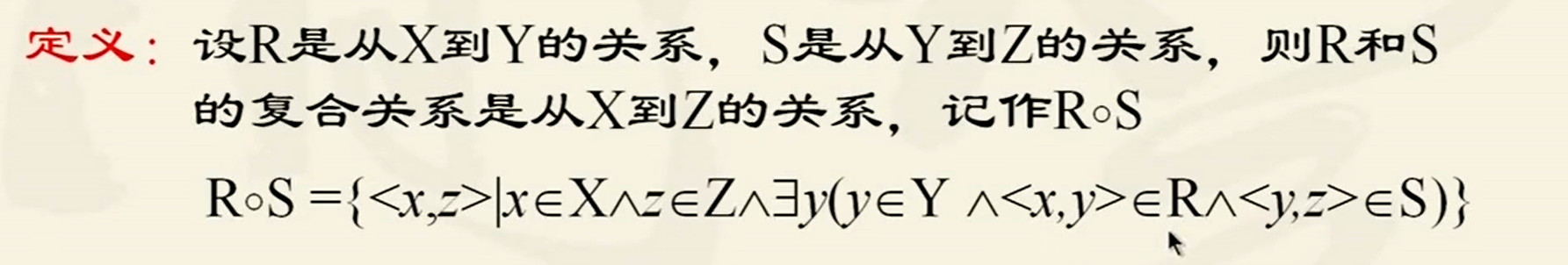关系符合的计算方法
有向图法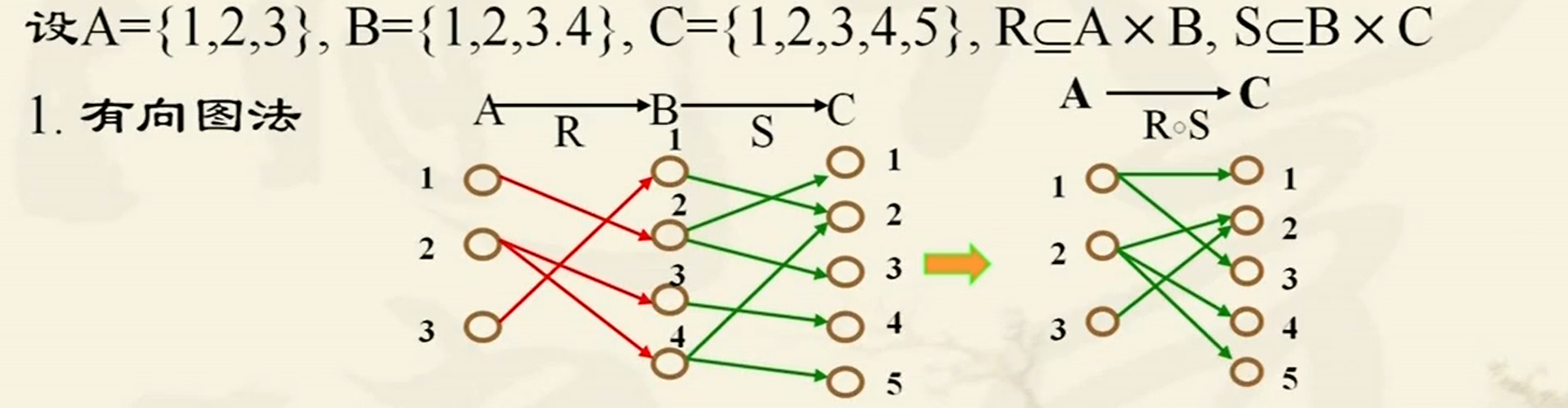枚举法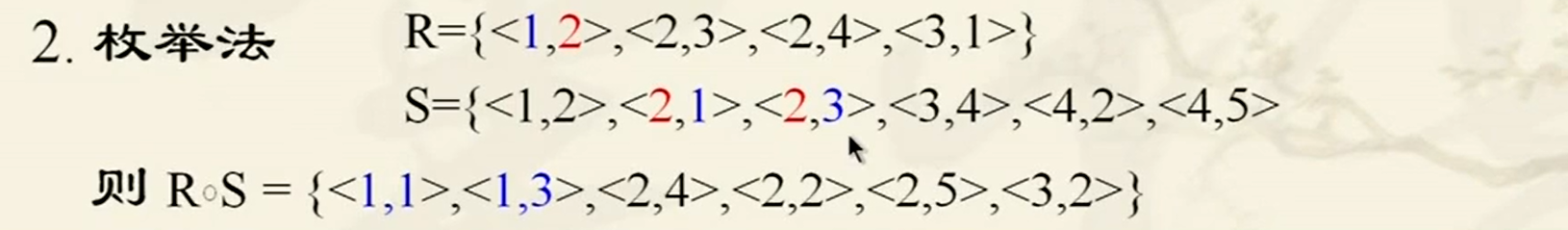谓词公式法
谓词公式法的计算过程就是函数的带入过程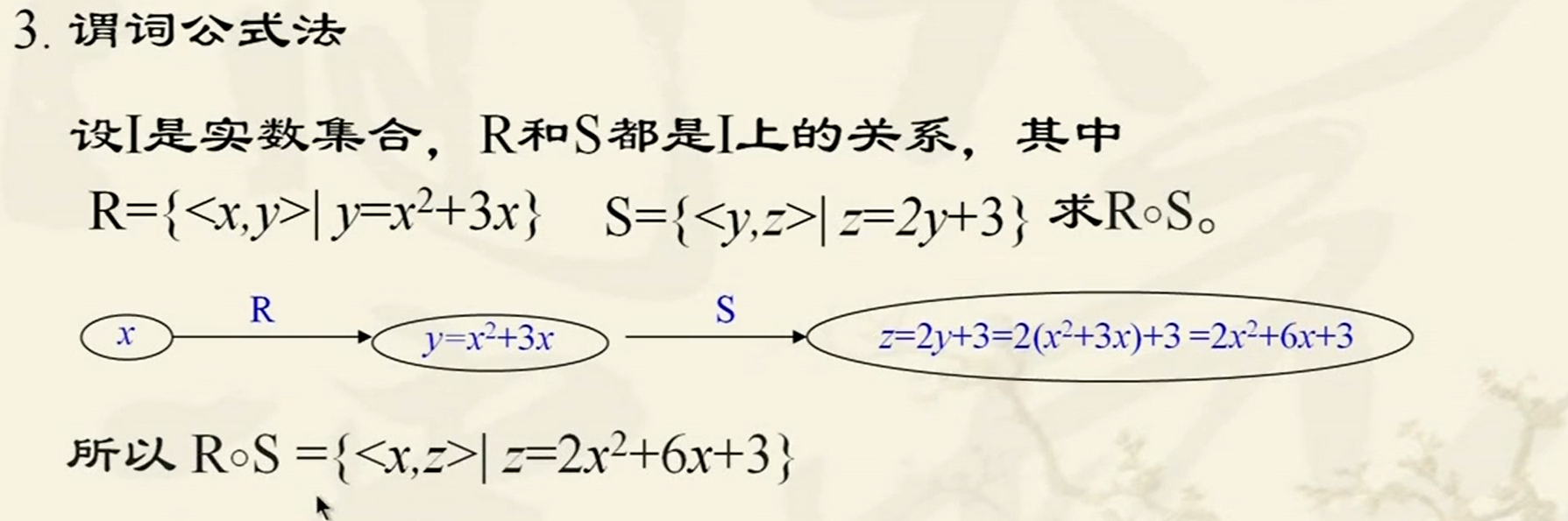矩阵法（扩展部分）
关系复合运算的性质
满足结合律，不满足交换律：

R

○

(

S

○

T

)

=

(

R

○

S

)

○

T

R○(S○T)=(R○S)○T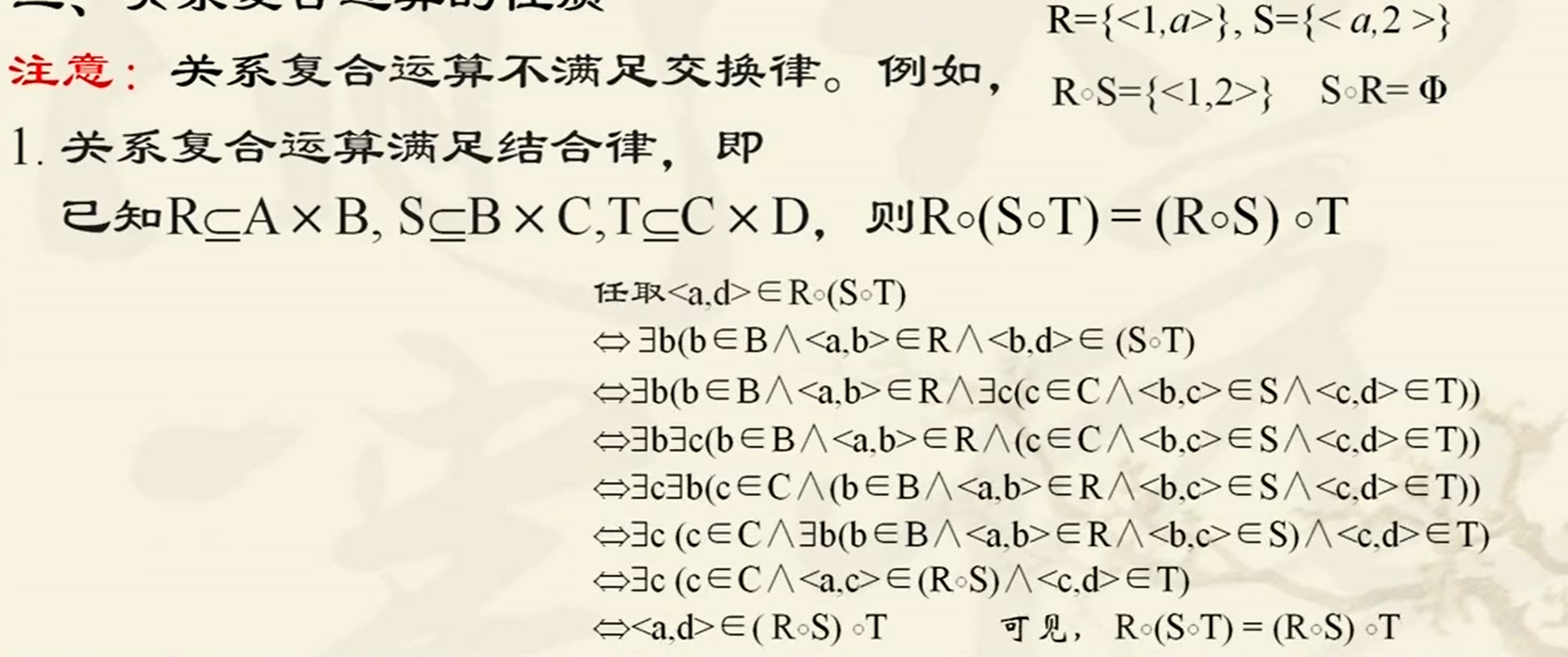R

○

(

S

∪

T

)

=

(

R

○

S

)

∪

(

R

○

T

)

R○(S \cup T)=(R○S) \cup (R○T)

R

○

(

S

∩

T

)

⊆

(

R

○

S

)

∩

(

R

○

T

)

R○(S \cap T)\subseteq (R○S) \cap (R○T)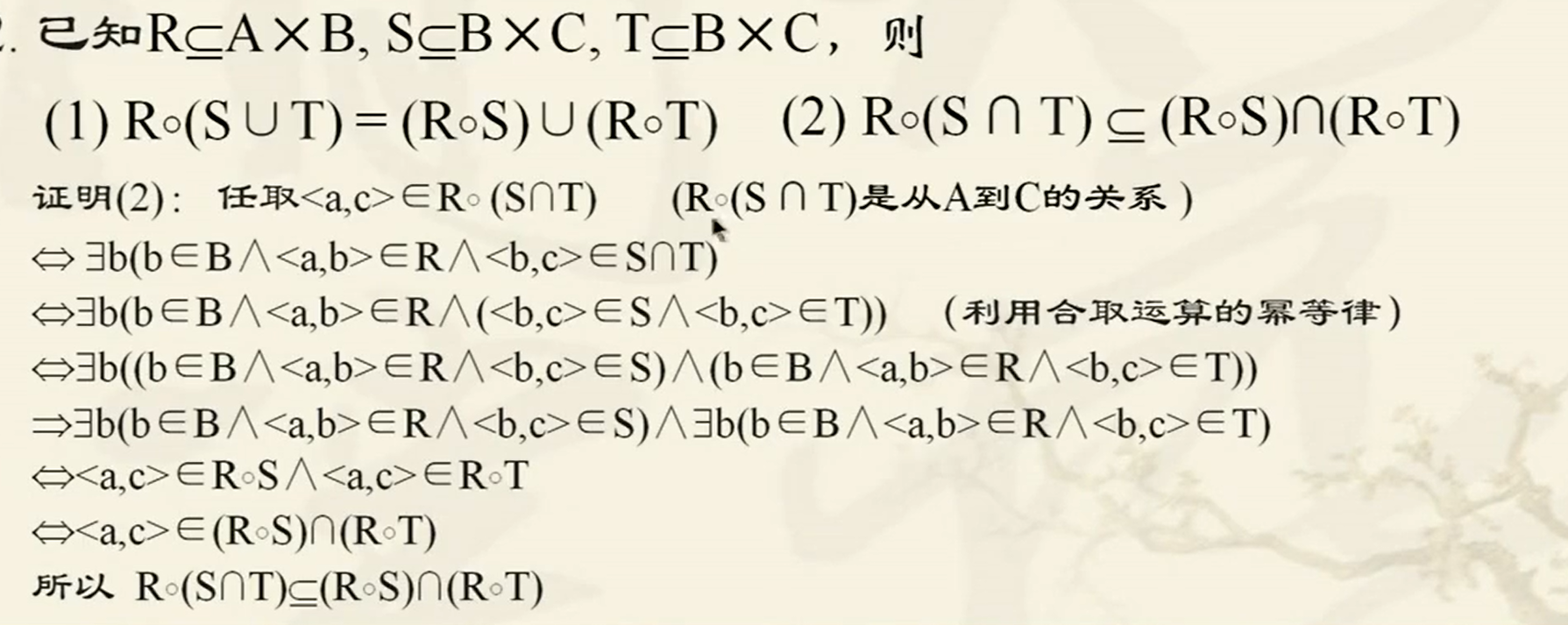R

○

I

B

=

I

A

○

R

=

R

R○I_B = I_A○R=R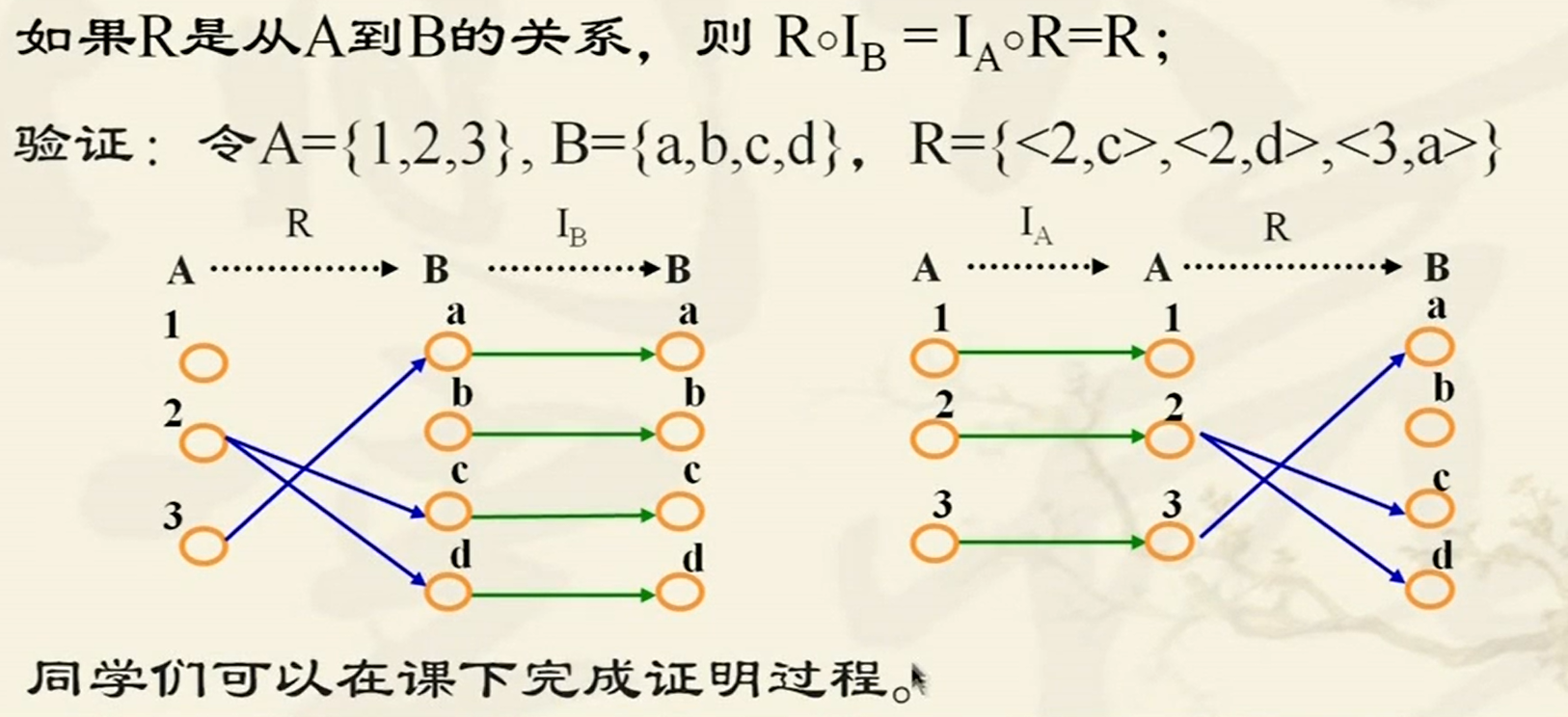关系的乘幂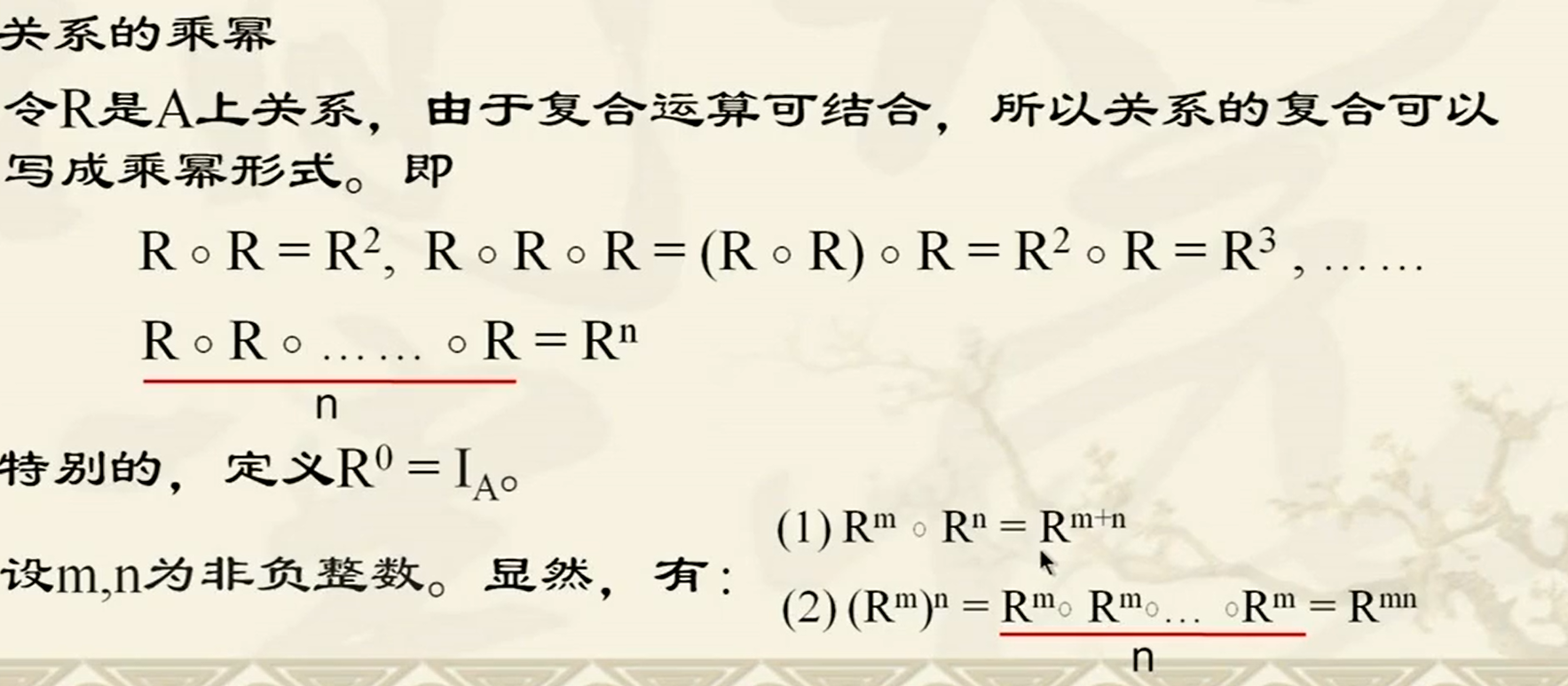I

A

I_A

代表的是

A

A

和自身的关系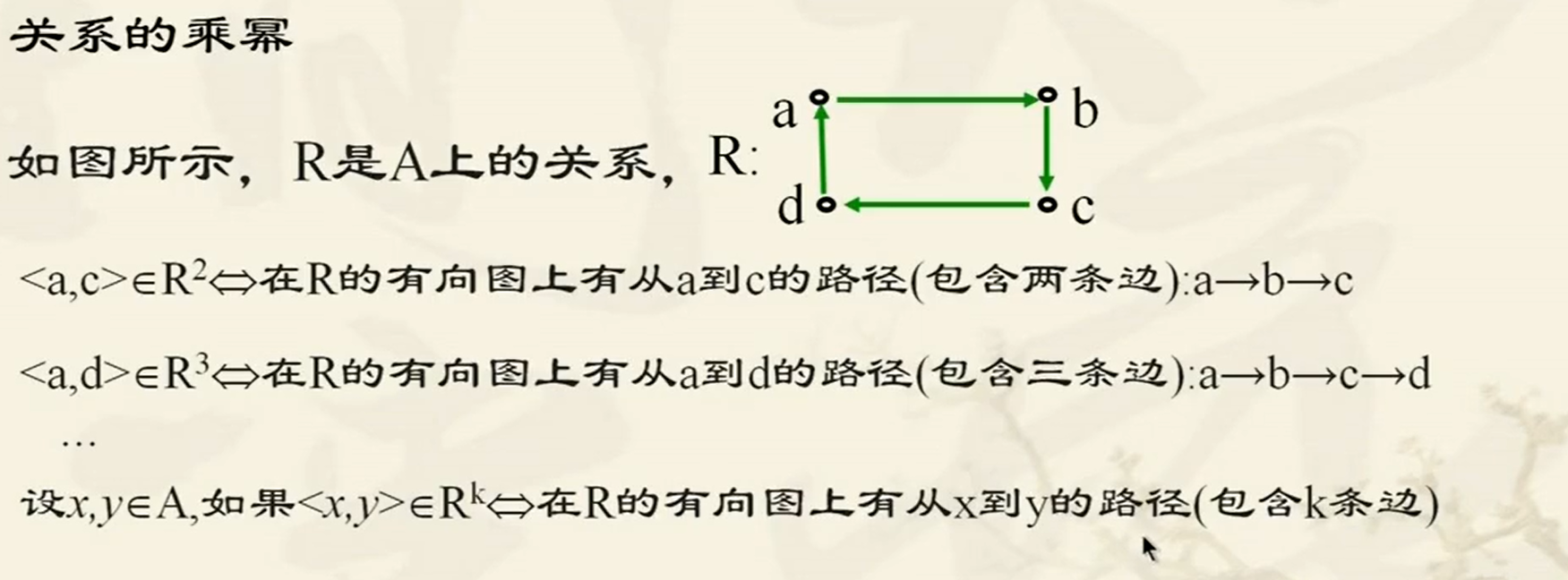关系的求逆运算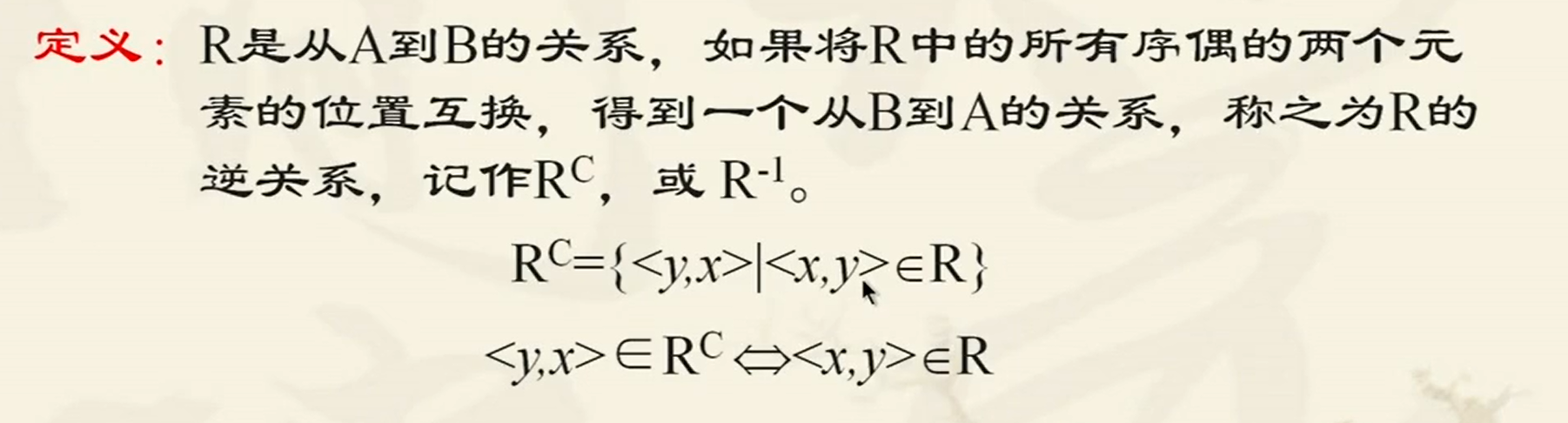关系求逆运算的计算方法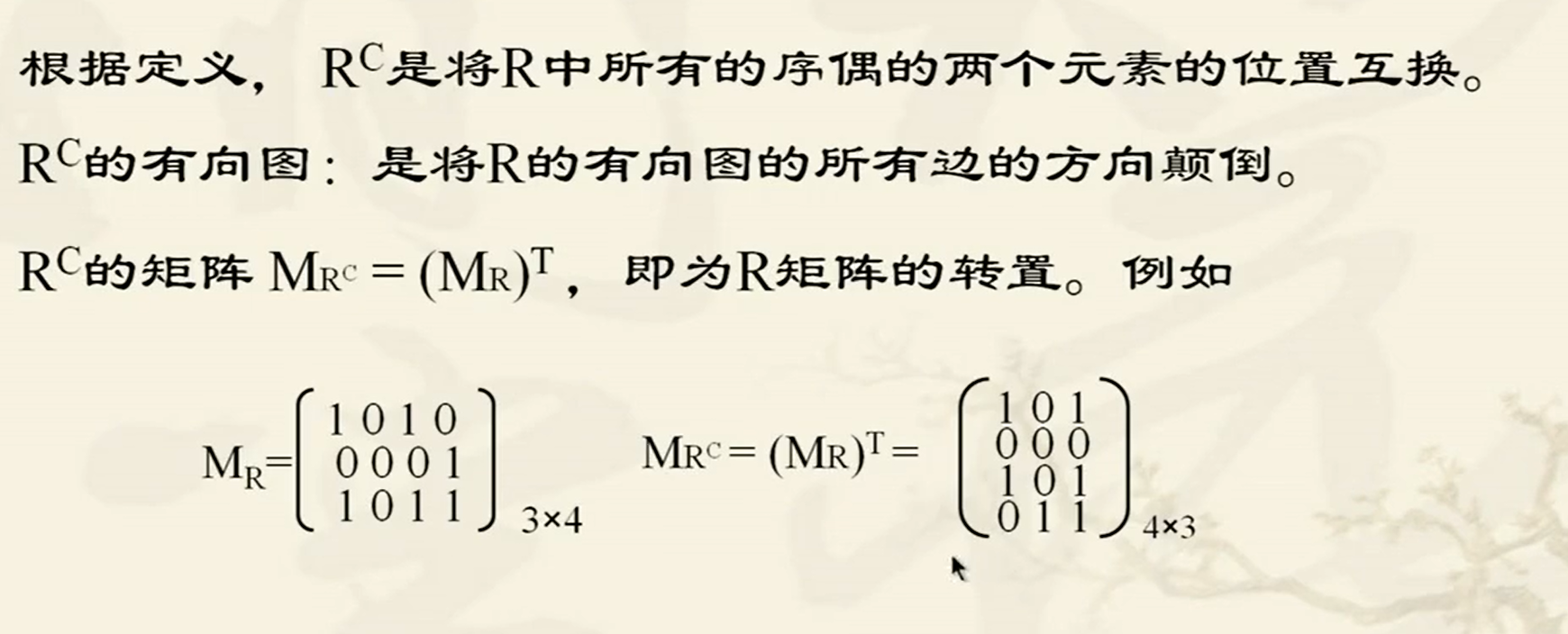求逆运算性质

(

R

C

)

C

=

R

(R^C)^C=R

(

R

∪

S

)

C

=

R

C

∪

S

C

(R \cup S)^C=R^C \cup S^C

(

R

∩

S

)

C

=

R

C

∩

S

C

(R \cap S)^C=R^C \cap S^C

(

R

−

S

)

C

=

R

C

−

S

C

(R - S)^C=R^C - S^C

(

R

⊆

S

)

≡

R

C

⊆

S

C

(R \subseteq S)\equiv R^C \subseteq S^C

(

∼

R

)

C

=

∼

R

C

(\sim R)^C=~\sim R^C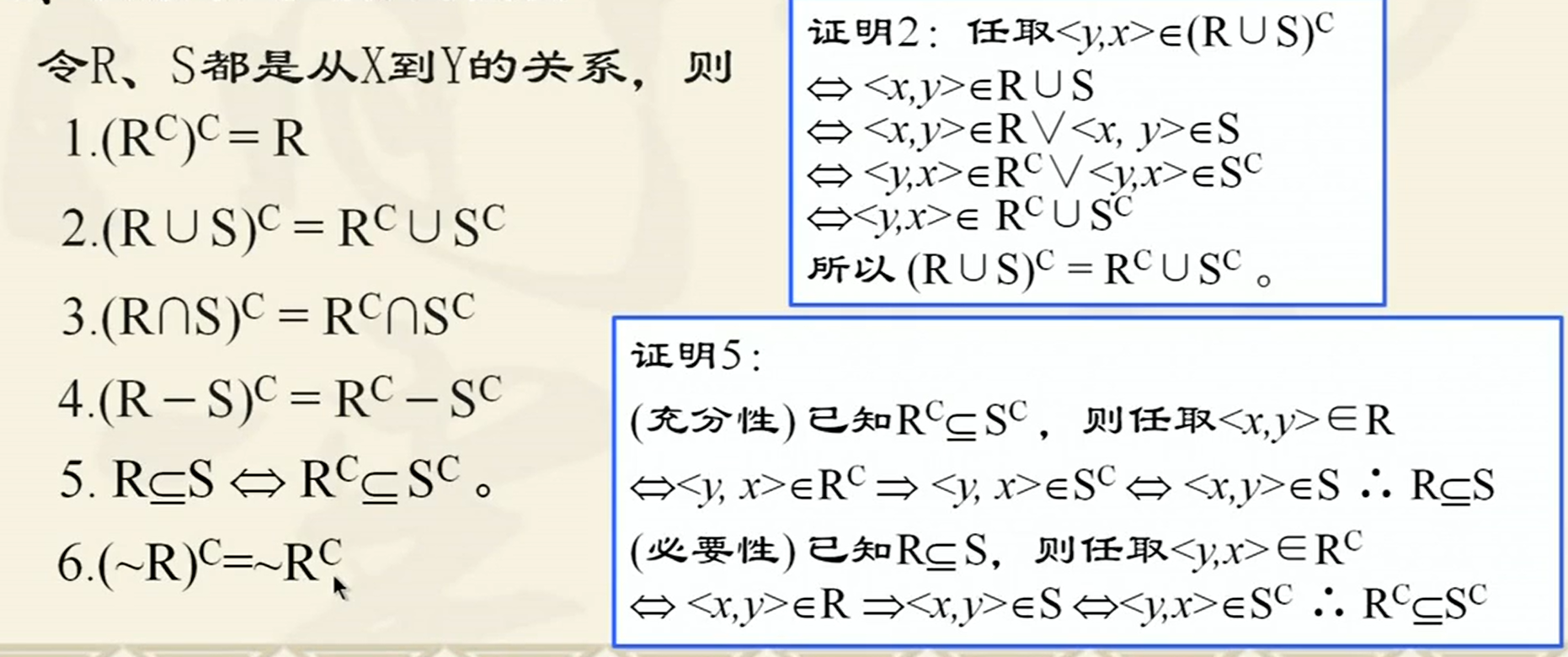(

R

○

S

)

C

=

S

C

○

R

C

(R○S)^C=S^C○R^C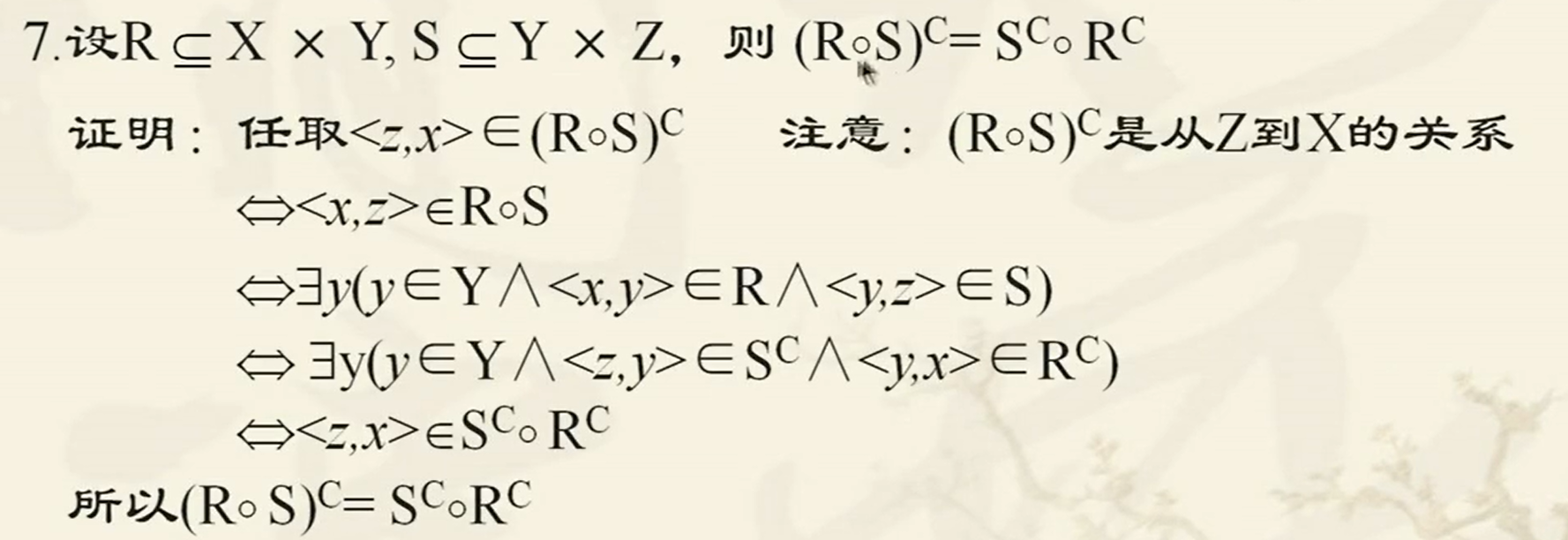i

f

f

R

C

=

R

iff ~R^C=R

，

R

R

是对称的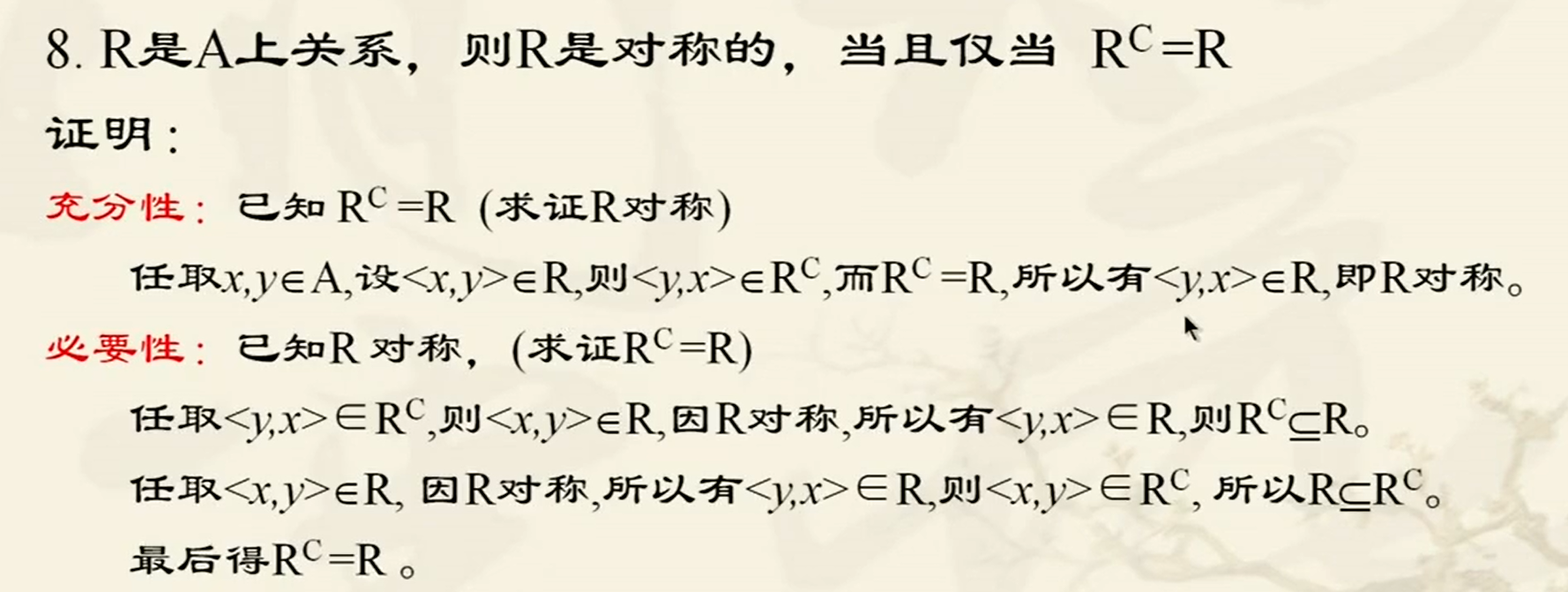展开全文算法 深度学习 机器学习
• 我尝试着编程实现了课本上集合与关系的相关内容，如集合的逆运算，复合运算，集合上关系性质判断与闭包运算等，基本判断方法均为定义法。 代码如下： #include<iostream> #include<vector> #...离散数学 C++
• 关系的运算 集合的运算 逆运算 例 R和R-1的关系关系的性质 复合运算 例 结合律 分配律 逆运算性质 ’R的n次幂 性质
• 1. 结合律与同一律 2. 关系复合运算的结合律的证明 3.关系复合运算的同一律的证明 4.关系复合运算的分配律的证明 5. 逆运算性质定律 ...
• 离散数学： 集合与关系-关系运算关系特有的运算关系复合 关系的逆 关系的幂 关系复合复合关系性质
• 一、关系数据结构及形式化定义 1、关系 关系模型的数据结构非常简单，只包含单一的数据结构——关系。... 笛卡儿积是域上的一种集合运算。 定义：给定一组域D1，D2，...，Dn，允许其中某些域是相同的，D...数据库
• 关系运算 & 等价关系和划分 关系运算 关系的合成 (1).设R1R_1R1​是A到B的关系,R2R_2R2​是B到C的关系,从A到C的合成关系记为R1R2R_1R_2R1​R2​定义为R1R2={<a,c>∣a∈A∧c∈C∧∃b(b∈B∧<a,b...
• 将输入/输出之间的逻辑关系用与/或/非的运算式表示就得到逻辑式。 逻辑图 用逻辑图形符号表示逻辑运算关系，与逻辑电路实现相对应。 波形图 将输入变量所有取值可能与对应输出按时间顺序排列起来画成时间波形。 ...
• 特别感谢原作者，感觉写得特别清晰，为方便日后... 关系模型的数据结构非常简单，只包含单一的数据结构——关系。在用户看来，关系模型中数据的逻辑结构是一张扁平的二维表。 1.1 域 域是一组具有相同数据类型值的...数据库
• 2.2 矩阵及其基本运算性质 如果你上过本科线性代数课程，你十有八九会对矩阵没有什么感觉，甚至对这么一个运算法则十分奇怪的东西感到厌恶。但我希望你今后能改变对矩阵、对线性代数的看法，不要让糟糕的教材和老师...
• 关系数据库关系数据模型关系是一个数学概念。 当把关系的概念引入到数据库系统作为数据模型的数据结构时，既有所限定和也有所扩充。 关系的数学定义例： 课程={离散,C语言…..}，学生={张三,李四…..} 笛卡儿积...数据库
• 关系数据库 一、关系数据结构及形式化定义 1.关系 单一的数据结构----关系 现实世界的实体以及实体间的各种联系均用关系来表示 逻辑结构----二维表 从用户角度，关系模型中数据的逻辑结构是一张二维表 建立在集合...数据库 大数据 笛卡尔积
• 函数的传统定义：设在某变化过程中有两个变量x、y，如果对于x在某一...函数的三种运算 函数的四则运算 复合运算 求逆运算函数的基本性质：单调性 有界性 奇偶性 周期性函数的近代定义：设A，B都是非空的数的集合，...
• * 复合运算性质 * 复合运算性质 * 复合运算性质 * 例 题 * 例 题 * 例 题 关系的基本运算 关系的集合运算 关系复合运算关系与逆关系 * 幂运算的定义 * 例 题 * 例 题 * 例 题 * 幂运算的性质 * 幂运算的...
• 运算 一、位运算概述 从现代计算机中所有的数据二进制的形式存储在设备中。即 0、1 两种状态，计算机对二进制数据进行的运算(+、-、 * 、/)都是叫位运算，即将符号位共同参与运算运算。相比在代码中直接使用(+...c++
• 要进行句法识别中基元之间关系的判别，首先要明确关系运算。 二元关系： 凡是一对对象之间的关系，都是二元关系。 二元关系的相关概念 （1）有序偶： 设用,y>表示一有序偶，它可以代表迪卡儿坐标，例如,3>...
• 补充知识点 ----- 异或的几条性质 取反运算 ----- ~ 左移运算符 ----- << 右移运算符 ----- >> 复合赋值运算符 总结 前言 想要学习位运算，首先我们应该了解什么是位运算、位运算中...java eclipse
• 图像基本运算概述型 图像基本运算的概述(Introduction) 图像基本运算的分类 点运算运算是指对一幅图像中每个像素...几何运算 几何运算就是改变图像中物体对象（像素）之间的空间关系。 从变换性质来分，几何...
• 从现代计算机找那个所有的数二进制的形式存储在设备总，即0,1两种状态，计算机对二进制数据进行的运算（加减乘除） 都是叫位运算，即将符号为共同参与运算运算。 然后我简单给出一个例子 int a = 35; int b = 47; ...编程语言
• 关系的定义域、值域、域 关系的逆运算及其性质 关系复合（合并）运算及其性质 关系的“限制”运算及其性质 集合在关系下的“像”及性质 关系的幂运算及其性质离散数学
• 运算（&、|、^、~、>>、<<） 1.位运算概述 从现代计算机中所有的数据二进制的形式存储在设备中。即0、1两种状态，计算机对二进制数据进行的运算(+、-、、/)都是叫位运算，即将符号位共同参与运算...java 补码 编程语言
• ## 图像基本运算

千次阅读 2017-01-09 18:00:55
图像处理是建立在各种算法基础上的处理方法，图像基本运算主要包括点运算、代数运算（加、减、乘、除）、逻辑运算（与、或、非）和几何运算（平移、镜像、旋转、缩放）。这些基本运算都具有十分重要的意义，如：...图像处理
• 主要探索了程度近似算子的乘积复合运算，定义了程度上、下近似算子的乘积运算，得到了其本质、基本结构与性质，为计算提出了宏观算法与结构算法，进行了算法分析与比较，得到了结构算法在算法时间、算法空间上优于......